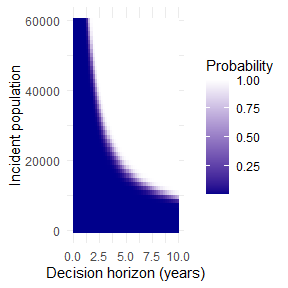# Plots of Value of Information measures

#### 2023-11-27

The voi package functions evppi(), evpi() and evsi() all return data frames in a “tidy” format with one row per VoI estimate. This allows plots to be produced and customised with little further effort using ggplot2.

This vignette demonstrates how to illustrate VoI analyses graphically, using an example EVSI results dataset chemo_evsi_or supplied with the voi package.

We also explain the use of the enbs() function to calculate and optimise the expected net benefit of sampling for a simple study design.

Knowledge of ggplot2 is required to be able to adapt these plots for your own analyses and communication needs, but plenty of learning resources are linked from the ggplot2 home page.

Some functions to create similar plots using base R graphics are supplied with the VoI Book.

library(voi)
library(ggplot2)
library(dplyr)

# Example EVSI dataset

The example dataset chemo_evsi_or included with the package gives the results of an EVSI analysis for the chemotherapy example model.

This object is a data frame with 15030 rows and three columns, giving the sample size per arm (n, from 50 to 1500), willingness-to-pay (k, from 10000 to 50000 pounds) and the corresponding EVSI (evsi).

head(chemo_evsi_or)
##    n   k evsi
## 1 50   0    0
## 2 50 100    0
## 3 50 200    0
## 4 50 300    0
## 5 50 400    0
## 6 50 500    0

Sidenote: The EVSI in this example is the expected value of a two-arm trial with a binary outcome of whether a patient experiences side-effects. The trial is only used to inform the log odds ratio of side effects between treatments, and information about the baseline risk of side effects is ignored. Source code for generating this dataset using the moment matching method in evsi() is provided here.

For reference, we also calculate the EVPI for the corresponding willingness-to-pay values, using output from probabilistic analysis of the decision model, as stored in the object chemo_cea_501 provided with the voi package.

evpi_df <- evpi(outputs = chemo_cea_501)

We also extract the values of the EVPPI (for the log odds ratio) into a data frame of the same format. These EVPPI values are already stored in chemo_evsi_or, because they had been calculated as a by-product of the moment matching method to calculate EVSI.

evppi_df <- attr(chemo_evsi_or, "evppi")

# EVSI curves

The following ggplot code produces curves of EVSI against willingness to pay, with different sample sizes shown by curves of different colours. The sample size is indicated by a colour gradient. The corresponding curves of EVPI, and EVPPI for the “focal” parameter of interest (the log odds ratio of side effects), are also shown for reference.

ggplot(chemo_evsi_or, aes(x=k, y=evsi, group=n, color=n)) +
geom_line() +
xlab("Willingness to pay (£)") + ylab("EVSI per person") +
geom_line(data=evpi_df, aes(x=k, y=evpi),
color="black", lwd=1.5, inherit.aes = FALSE) +
geom_line(data=evppi_df, aes(x=k, y=evppi),
color="darkblue", lwd=1.5, inherit.aes = FALSE) +
labs(color="Sample size") +
xlim(0,52000) +
annotate("text",x=50000,y=125,label="EVPI",hjust=0) +
annotate("text",x=50000,y=107,label="EVPPI",color="darkblue",hjust=0) +
annotate("text",x=50000,y=50,label="EVSI",color="darkblue",hjust=0)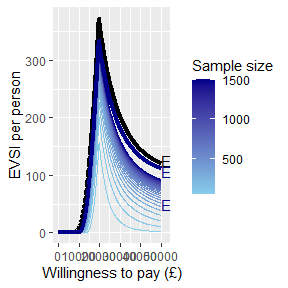Or we can adapt the plot to only show a limited number of sample sizes, shown in a discrete legend, and customise the colour range.

n_use <- c(250, 500, 1000)
chemo_evsi_or %>%
filter(n %in% n_use) %>%
ggplot(aes(x=k, y=evsi, group=n, color=n)) +
geom_line(lwd=1.5) +
scale_colour_binned(breaks=n_use,
low = "gray80", high = "gray20",
guide=guide_legend(title="Sample size",
reverse=TRUE)) +
xlab("Willingness to pay (£)") + ylab("EVSI (per person)") +
geom_line(data=evppi_df, aes(x=k, y=evppi),
color="darkblue", lwd=1.5, lty=3, inherit.aes = FALSE) +
annotate("text", x=50000, y=130, col="darkblue", label="EVPPI", hjust=1)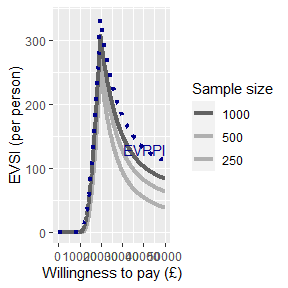In a similar way, we can produce curves of EVSI against sample size, with different willingness-to-pay (WTP) values shown by curves of different colours. To reduce visual clutter, only a limited number of willingness-to-pay values are illustrated. This plot shows how the EVSI converges to the (WTP-dependent) EVPPI for the focal parameter (log odds ratio of side effects) as the sample size increases.

chemo_evsi_or %>%
filter(k %in% seq(10000,50000, by=1000)) %>%
ggplot(aes(x=n, y=evsi, group=k, color=k)) +
geom_line() +
xlab("Sample size") + ylab("EVSI per person") +
labs(color="Willingness-to-pay (£)")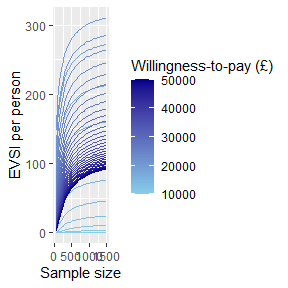# Expected net benefit of sampling

The voi package includes a function enbs() to obtain the expected net benefit of sampling for a proposed study of individual participants, given estimates of EVSI. See the help page enbs() for full documentation for this function.

The enbs() function supposes that the costs of the study include a fixed setup cost (costs_setup), and a cost per participant recruited (costs_pp). (Note that if n describes the sample size per arm in a two-arm study, costs_pp is actually the cost of recruiting two people, one in each arm.) To acknowledge uncertainty, a range of costs may be supplied as two numbers. These are assumed to describe a 95% credible interval for the cost, with the point estimate given by the midpoint between these two numbers.

Additional required information includes the size of the population affected by the decision (pop), and a discount rate (disc, by default 0.035) to be applied over a time horizon time. These may be supplied as vectors, in which case ENBS is calculated for all potential combinations of values (see below for an example).

Here is an example of calling enbs(). The result is a data frame giving the sample size (n), willingness-to-pay (k), and corresponding ENBS (enbs). The standard deviation (sd) describes the uncertainty about the ENBS estimate due to the uncertain costs. pce is the probability that the study is cost-effective (i.e. has a positive net benefit of sampling). The other components of this data frame are related to the optimal sample size (as discussed below)

nbs <- enbs(chemo_evsi_or,
costs_setup = c(5000000, 10000000),
costs_pp = c(28000, 42000),
pop = 46000,
time = 10)
nbs %>%
filter(k==20000) %>%
head()
##     n     k     enbs      sd pce ind  enbsmax nmax nlower nupper
## 1  50 20000 48163756 1262191   1 201 84091443  450    250    750
## 2 100 20000 63770725 1298075   1 201 84091443  450    250    750
## 3 150 20000 72116178 1355775   1 201 84091443  450    250    750
## 4 200 20000 77115463 1432655   1 201 84091443  450    250    750
## 5 250 20000 80233182 1525819   1 201 84091443  450    250    750
## 6 300 20000 82172795 1632483   1 201 84091443  450    250    750

The expected net benefit of sampling for a given willingness-to-pay can then be illustrated by filtering the nbs dataframe to this willingness-to-pay value, then passing it to a simple ggplot.

nbs %>%
filter(k==20000) %>%
ggplot(aes(x=n, y=enbs)) +
geom_line() +
xlab("Sample size") + ylab("Expected net benefit of sampling") +
scale_y_continuous(labels = scales::dollar_format(prefix="£"))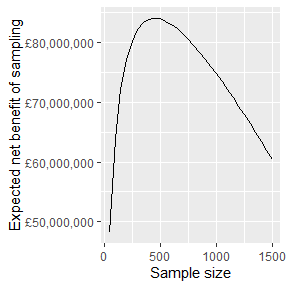Note the scales::dollar_format trick for showing large currency values tidily in the axis labels.

The same colouring techniques used above (using a group aesthetic, with scale_colour_gradient) might also be used to illustrate the dependence of the ENBS curve on the willingness-to-pay.

Uncertainty around the ENBS can be illustrated by plotting quantiles of the ENBS alongside the point estimates. These quantiles can be derived from the point estimate (enbs) and the standard deviation (sd) stored in the data frame that we called nbs, assuming a normal distribution.

nbs %>%
filter(k==20000) %>%
mutate(q975 = qnorm(0.975, enbs, sd),
q75 = qnorm(0.75, enbs, sd),
q25 = qnorm(0.25, enbs, sd),
q025 = qnorm(0.025, enbs, sd)) %>%
ggplot(aes(y=enbs, x=n)) +
geom_ribbon(aes(ymin=q025, ymax=q975), fill="gray80") +
geom_ribbon(aes(ymin=q25, ymax=q75), fill="gray50") +
geom_line() +
xlab("Sample size") + ylab("Expected net benefit of sampling") +
scale_y_continuous(labels = scales::dollar_format(prefix="£")) +
annotate("text",x=1100,y=85000000,label="95% credible interval") +
annotate("text",x=1250,y=70000000,label="50% credible interval")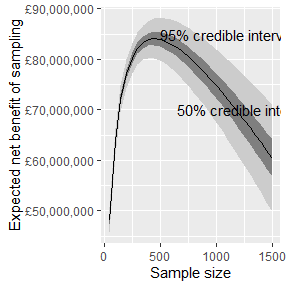# Optimal sample size

The enbs() function also estimates the optimal sample size for each willingness-to-pay value. That is, the sample size which gives the maximum expected net benefit of sampling. The optimal sample size can be estimated using two alternative methods.

1. A simple and fast method: just pick the highest ENBS from among the sample sizes included in the evsi object that was supplied to enbs().

2. A more sophisticated (but slower) method uses nonparametric regression to smooth and interpolate the ENBS estimates. This is useful if the EVSI has only been computed for a limited number of sample sizes, or if the EVSI estimates are noisy (which may happen for the regression-based method). We might judge that the ENBS is a smooth function of sample size, that we could estimate by regression on the estimates that have been computed.

## Smooth interpolation

Here is a demonstration of how the regression smoothing method works.

• Suppose we have computed EVSI (hence ENBS) for a limited set of sample sizes, say eight, from 100 to 800 by 100, and for a specific willingness to pay (and population size, horizon and discount rate).

• The function enbs_opt is used to create from this a smoothed and interpolated dataset (here called preds) that contains 701 ENBS estimates, for every integer from 100 to 800. The maximum ENBS can then be found by searching through this interpolated dataset.

nbs_subset <- nbs %>%
filter(k==20000, n %in% seq(100,800,by=100))
eopt <- enbs_opt(nbs_subset, smooth=TRUE, keep_preds=TRUE)

The enbs_opt function returns the maximum ENBS (enbsmax), the optimal sample size (nmax) and an interval estimate (nlower to nupper) defined by the lowest and highest sample size that have ENBS within 5% of the optimum.

eopt
##     ind  enbsmax nmax nlower nupper
## 393 201 83950193  492    239    788

The full interpolated dataset is also returned as the "preds" attribute.

preds <- attr(eopt, "preds")
head(preds, 2)
##     n     enbs ind  enbsmax nmax nlower nupper
## 1 100 63987440 201 83950193  492    239    788
## 2 101 64128147 201 83950193  492    239    788

In this graph, the eight pre-computed ENBS values are shown as points, and the 701 smoothed/interpolated values are shown on a line.

ggplot(nbs_subset, aes(x=n, y=enbs)) +
geom_point() +
geom_line(data=preds) +
xlab("Sample size") + ylab("Expected net benefit of sampling") +
scale_y_continuous(labels = scales::dollar_format(prefix="£")) +
geom_vline(data=eopt, aes(xintercept=nlower), col="blue", lty=2) +
geom_vline(data=eopt, aes(xintercept=nmax), col="blue", lty=2) +
geom_vline(data=eopt, aes(xintercept=nupper), col="blue", lty=2) +
geom_hline(data=eopt, aes(yintercept=enbsmax), col="blue", lty=2)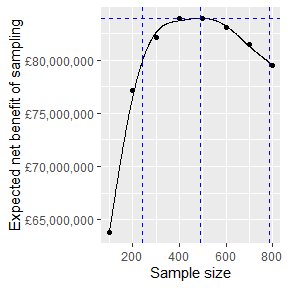The maximum, and the range of sample sizes whose ENBS is within 5% of this maximum, are shown as blue dotted lines.

Technical note: this uses the default spline regression model s() from the gam function in the mgcv package. The flexibility of the fitted model can be configured by setting the smooth_df argument to either enbs or enbs_opt. This is passed as the k argument to the s() function, and defaults to 6 (or the number of sample sizes minus 1, if this is lower than 6).

enbs_opt assumes a single willingness-to pay (and population size, etc). In practice you do not need to use enbs_opt, unless you want to check the goodness-of-fit of the regression that is fitted. Instead you can just call enbs(..., smooth=TRUE), and this will determine the optimal sample size, using smoothing, for each of multiple willingness-to-pay values (see the nbs_smoothed object below for an example).

In this example, there are a wide range of sample sizes within 5% of the optimum. In practice, the cheapest study, the one at the bottom of this range, might still be deemed acceptable to decision-makers. There is still a lot of unacknowledged uncertainty in this analysis, including about the costs, incident population size, decision horizon, and computational uncertainty in the per-person EVSI estimate. The location of the “exact” maximum of the curve of ENBS versus sample size might be considered a minor issue in the context of these other uncertainties.

## Curve of optimal sample size

For convenience, the enbs() function also returns, as the "enbsmax" attribute, a data frame with one row per willingness-to-pay (k), giving the optimal ENBS (enbsmax) the optimal sample size (nmax) and an interval estimate for the optimal sample size (nlower to nupper). An extract is shown here.

attr(nbs, "enbsmax") %>%
filter(k %in% seq(20000,50000,by=10000))
##   ind    enbsmax nmax nlower nupper     k
## 1 201 84091443.3  450    250    750 20000
## 2 301 24976901.6  450    350    600 30000
## 3 401  7291455.8  400    350    500 40000
## 4 501   406604.1  400    400    400 50000

This allows a plot to be drawn of the optimal sample size against willingness to pay, with an interval estimate defined by this range of “95% optimal” sample sizes. This is compared here for the two methods of determing the optimum: with and without smoothing.

p1 <- attr(nbs, "enbsmax") %>%
ggplot(aes(y=nmax, x=k)) +
geom_ribbon(aes(ymin=nlower, ymax=nupper), fill="gray") +
geom_line() +
ylim(0,800) +
xlab("Willingness to pay (£)") + ylab("Optimal sample size") +
ggtitle("Unsmoothed")
nbs_smoothed <- enbs(chemo_evsi_or,
costs_setup = c(5000000, 10000000),
costs_pp = c(28000, 42000),
pop = 46000, time = 10, smooth=TRUE)
p2 <- attr(nbs_smoothed, "enbsmax") %>%
ggplot(aes(y=nmax, x=k)) +
geom_ribbon(aes(ymin=nlower, ymax=nupper), fill="gray") +
geom_line() +
ylim(0,800) +
xlab("Willingness to pay (£)") + ylab("Optimal sample size") +
ggtitle("Smoothed")
gridExtra::grid.arrange(p1, p2, nrow=1)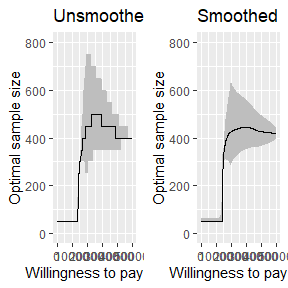# Probability of a cost-effective trial

Given the uncertainty about the incident population size and decision time horizon, we might want to show how the results depend on these. This might be done using a heat map.

The next plot selects a specific willingness to pay (£20,000) and plots the probability that a study with a sample size of 400 is cost effective, i.e. the probability that the expected net benefit of sampling is positive. This is compared on a grid defined by a range of population sizes from 0 to 60000, and a range of decision horizon times from 0 to 10.

nbs <- enbs(chemo_evsi_or %>% filter(k==20000, n==400),
costs_setup = c(5000000, 10000000),
costs_pp = c(28000, 42000),
pop = seq(0,60000,length.out=50),
time = seq(0,10,length.out=50))
ggplot(nbs, aes(y=pop, x=time, fill=pce)) +
geom_raster() +
labs(fill="Probability") +
theme_minimal()